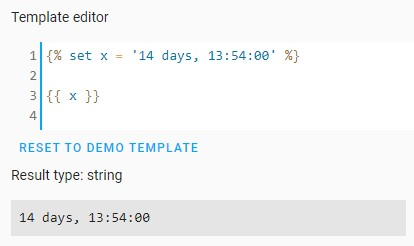# Why the heck is there no timedelta for easier date math?

Currently, if you want to do some simple date math, like add or subtract 7 days from today’s date, you have to go through arithmetic gymnastics involving conversions to and from timestamps. It would be far simpler if python’s `timedelta` were available for templating purposes.

Subtracting 7 days from today’s date would be just this:

``````{% set the_past =  now() - timedelta(days=7) %}
``````

The date and time in 12 hours:

``````{% set the_future =  now() + timedelta(hours=12) %}
``````

TimeDelta

Yes! That would be a great addition.

i don’t even need to read, instant vote

A bonus would be to include python’s `date` so we can define a datetime object like this:

``````{% set birthday = date(2000, 5, 23) %}
``````

as opposed to the current back-flips involving `strptime` or using the long-winded:

``````now().replace(year=2000, month=5, day=23, hour=0, minute=0, second=0, microsecond=0)
``````
1 Like

and if we add timedeltas, make the custom filters work off them. Might as well extend relative time to work in the future as well. There’s about 90 kajillion things that need to be added to make time calculations better in templates.

1 Like

Would you envision timedelta() supporting templates?

e.g. `"{{ now() - timedelta( days = states('input_number.days_ago') ) }}`

Sounds good to me.

There’s a PR in the pipeline to implement `timedelta` however it only accepts values in seconds (as opposed to weeks, days, minutes, etc).

Seconds is fine with me. Not ideal but better.

Better than nothing but only a partial implementation of python’s `timedelta`.

Yes, but I mean as long as you can do math in the timedelta then. There is no harm.
I mean instead of days=7 just do 7*86400.
But two times a year that will fail.

Ok I withdraw my previous comment.
Yes full timedelta is better.

Don’t know how much extra that is in implementation, but since the function exists in python I assume it’s minimal.

It seems like timedelta is completely implemented now: https://github.com/home-assistant/home-assistant.io/pull/14384/files.

The PR is merged.

Guys,

Maybe I’m missing it here but I’m having a very hard time with the Home Assistant timedelta sensor. I’m trying to calculate a “bandwidth per day” value by using the time left in my billing cycle and the remainder of my data cap but for some reason the Home Assistant timedelta sensor I created doesn’t contain the same attributes as the timedelta python object.

I was referencing the timedelta python page and it shows that timedelta objects have attributes for days, seconds and microseconds so I implemented this:

``````      sparklight_usage_per_day_left:
friendly_name: Sparklight Usage Per Day Left
value_template: >
{{ (states('sensor.sparklight_usage_remaining') / (state_attr('sensor.sparklight_billing_days_left', 'days') + state_attr('sensor.sparklight_billing_days_left', 'seconds')/86400)) | round(2) }}
``````

It doesn’t work, and through the template page I found that `{{ states('sensor.sparklight_billing_days_left') }}` results in a string like: `14 days, 13:54:00` and I cannot get attributes for `days`, `seconds`, nor `microseconds` from the sensor. I tried piping to float and int and that results in zero. Is there an easy way to do what I’m trying to do?

Thanks,
-Greg

An entity’s State value is always a string type. Therefore I don’t understand how this:

``````{{ states('sensor.sparklight_billing_days_left') }}
``````

can be expected to report a value whose type is timedelta.

Or, because of the new support for native types, were you expecting this string:

``````14 days, 13:54:00
``````

to be inferred as a timedelta type?

I tried it in the Template Editor and it definitely doesn’t perform that kind of type conversion:I guess I was – or at least with attributes that represented the constituents of the timedelta. I can think of two ways to do this but both seem very non-pythonic:

1. I can duplicate the code for calculating the timedelta in the template sensor where I need it because then the python timedelta object contains the days/seconds attributes (`billing_time_left.days`, and `billing_time_left.seconds`). I did this in the template tool first to prove out the functionality but I was hoping to easily re-use the sensor values in the same way.
``````{%- set today = now().replace(tzinfo=None, microsecond=0) -%}
{%- set billing_day = strptime('{}-{}-21'.format(today.year, today.month),'%Y-%m-%d') -%}
{%- if as_timestamp(billing_day) - as_timestamp(today) <= 0 -%}
{%- if today.month == 12 -%}
{%- set billing_day = strptime('{}-01-21'.format(today.year+1),'%Y-%m-%d') -%}
{%- else -%}
{%- set billing_day = strptime('{}-{}-21'.format(today.year, today.month+1),'%Y-%m-%d') -%}
{%- endif -%}
{%- endif %}
{%- set billing_time_left = billing_day - today -%}

If today is: {{ today }}
Billing day is: {{ billing_day }}
Bandwidth left: {{ (350 - (states('sensor.wan_monthly_data_in')|float + states('sensor.wan_monthly_data_out')|float)/1e9)|round(4) }}
Time left: {{ billing_time_left }}
Bandwidth left per day: {{ (((350 - (states('sensor.wan_monthly_data_in')|float + states('sensor.wan_monthly_data_out')|float)/1e9)|round(4))/(billing_time_left.days + billing_time_left.seconds/86400)) | round(2) }}GB/day
``````

This code works all together, and produces:

``````If today is: 2020-12-06 10:56:44
Billing day is: 2020-12-21 00:00:00
Bandwidth left: 291.2344
Time left: 14 days, 13:03:16
Bandwidth left per day: 20.02GB/day
``````
1. Alternatively, I can decompose the string into separate `days` and `timestamp` values and manually calculate the floating point number of days remaining.

It just feels dirty to copy the entire block of code into a second sensor just to recalculate the timedelta object so I can use it again for another purpose.

Looking for guidance on how best to handle this. Also, I know you guys are much more efficient at logic blocks than I am so if you have suggestions on ways to tighten it up, I’m all ears!